# An Insight into the Concept of Normal Stress

Normal stress describes the element-level intensity of internal normal force, and it is defined as the normal force exerted by two adjacent elements on each other, divided by the area on which the force acts.

We need a way of describing forces at the level of elements.Force per area or stress is an effective way of describing forces on elements, since the force is essentially uniform on neighboring, identical, infinitesimal elements.

1. Since the forces on small, neighboring elements are equal, force per area describes element forces. We know that the forces acting on small neighboring elements are equal. Say the force on an element of area A is P. Then, if four of those elements are combined into one with area 4ΔA, its force must be 4ΔP. Note that the force per area
4ΔP/4ΔA=ΔP/ΔA
is the same. We can summarize all such combinations of element areas and forces by saying that the force per area ΔP/ΔA is locally uniform.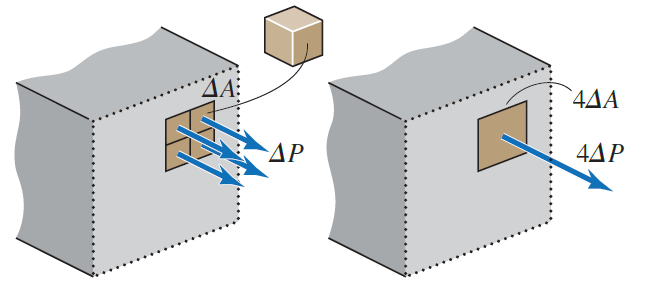We define stress, , as the force per area on an element. Stress alone, without reference to force or area individually, describes forces acting on elements.
σ=ΔP/ΔA
Stress has units of
Force/Area:
N/m^2 = Pa or lb/in^2 = psi

2. Draw stresses on an exposed internal surface of a body or just acting on a single element. Sometimes we depict stress by showing it acting on the surface of an element still embedded in the rest of the body (as above). Like forces, which obey Newton’s 3rd Law, an equal and opposite stress acts on an element in the adjoining body across their common surface.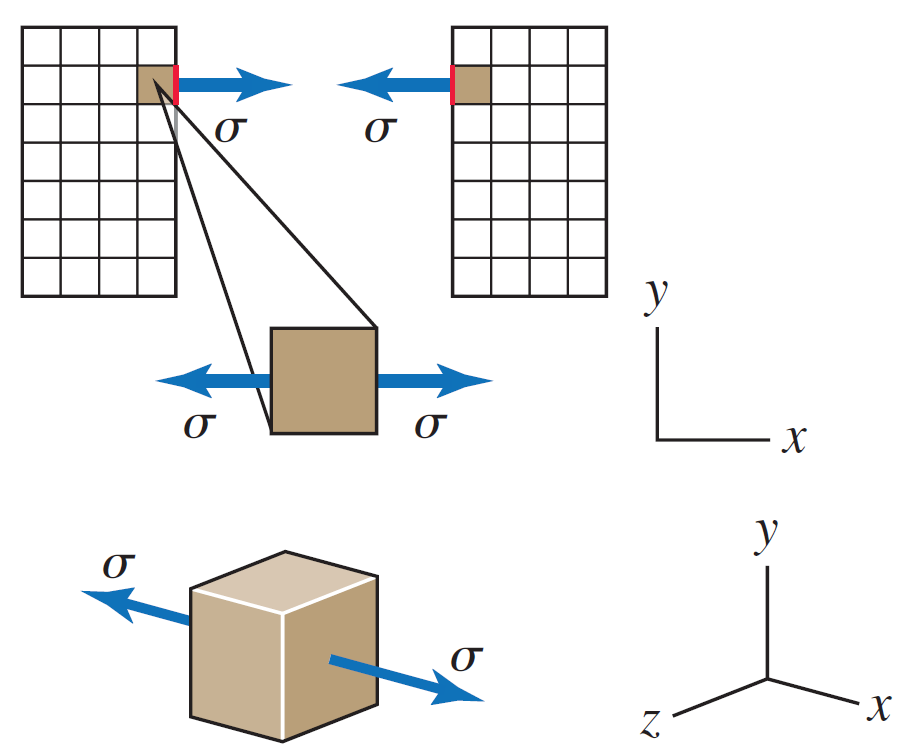Sometimes we depict stress by drawing only an element, in 2-D or 3-D, and the stresses on its faces. Usually, the element is aligned with x-y-z axes. Notice that the element is in equilibrium, because the two forces on it, each equal to the stress times the element area, are equal and opposite.

3. Be aware that stress on an element can be very complex. We will see that stress, even on a single element, can become complicated. The stress considered so far is normal stress: the force acts perpendicularly (normally) to the element face.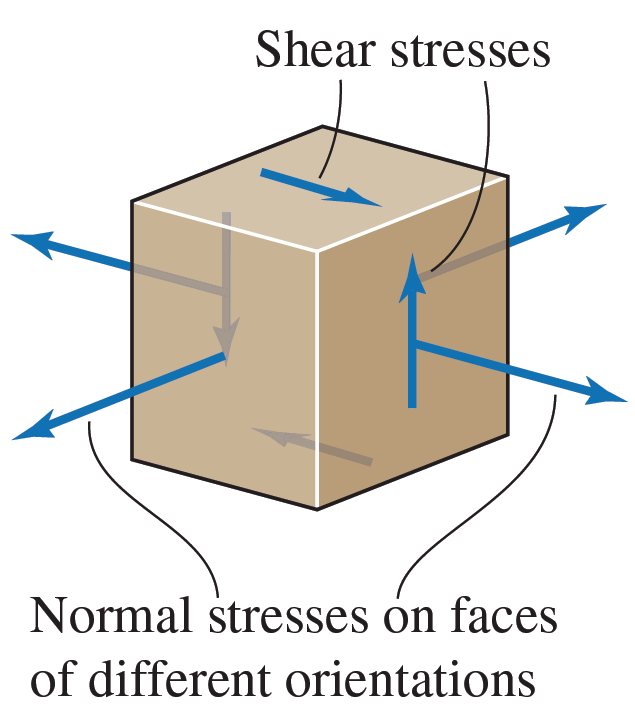4. Stresses often vary across a surface cut through a body, but they are statically equivalent to the internal loads acting on the surface. In general, stress will vary from element to element gradually across a surface. But, the element forces, when combined, are statically equivalent to the internal force and moment. That is why internal loads are sometimes referred to as stress resultants.
Mechanics of Materials addresses commonly occurring situations in which the distribution of stress across an internal surface can be found from the internal load and the shape of the surface.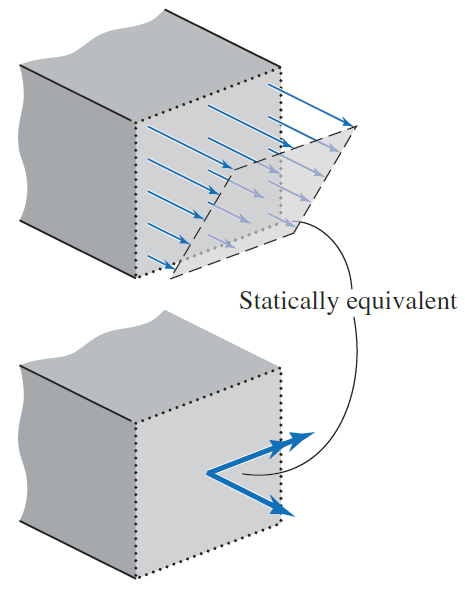5. In special circumstances, the normal stress may be uniform across an entire surface. Imagine forces of magnitude P were applied to a deformable rectangular sheet of rubber. Lines have been drawn on the sheet to show the deformation of individual elements when equal and opposite forces are applied at the two dots. Here the sheet is deformed while forces act. Focus on the elements at two cross-sections: S1 and S2.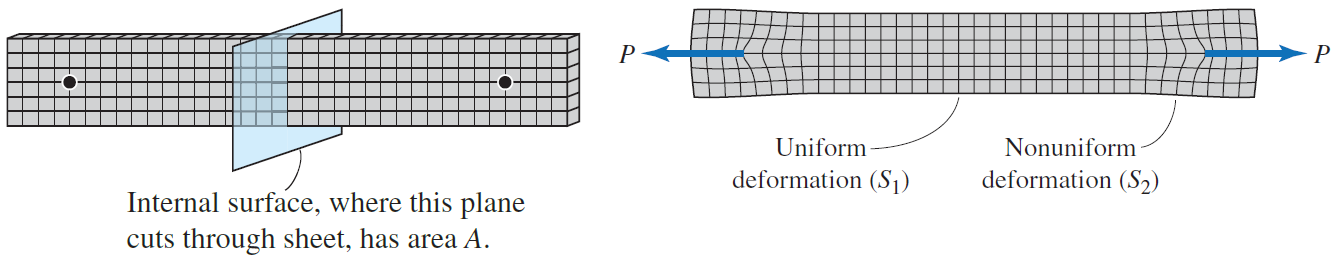In a region such as S2, the deformation is nonuniform, so the stress will be nonuniform.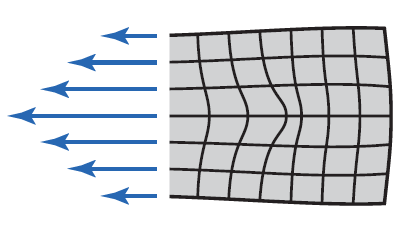Nonuniform stresses at region S2
Because the internal force on the sheet is the sum of the forces across all the elements, the average normal stress, σavg, can be found even if the stress is nonuniform:
σavg=P/A
We observe that the elements deform identically in the central portion of the sheet (S1), away from the applied forces.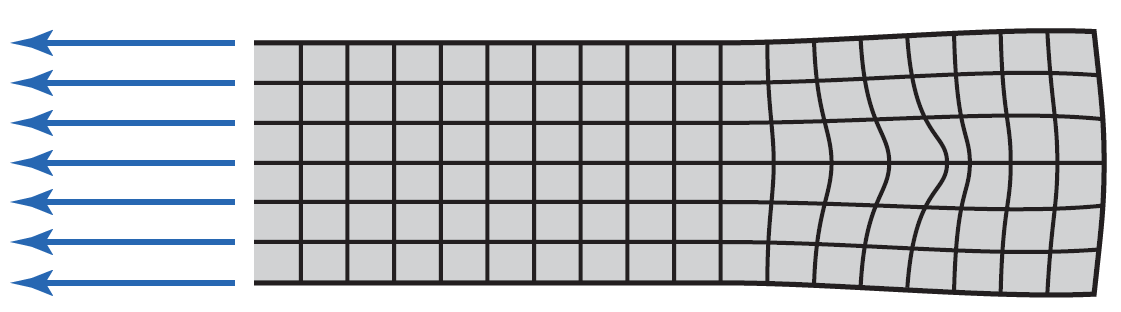Uniform stresses at region S1
Since the material is uniform, the forces on all elements must be equal. So, in the central portion of the bar where the element forces are uniform and the stress at each point is also equal to the average stress:
σavg=P/A
6. The local normal stress is  uniform and equal to P/A under certain conditions. The normal stress is uniform (so σ = P/A), under these conditions: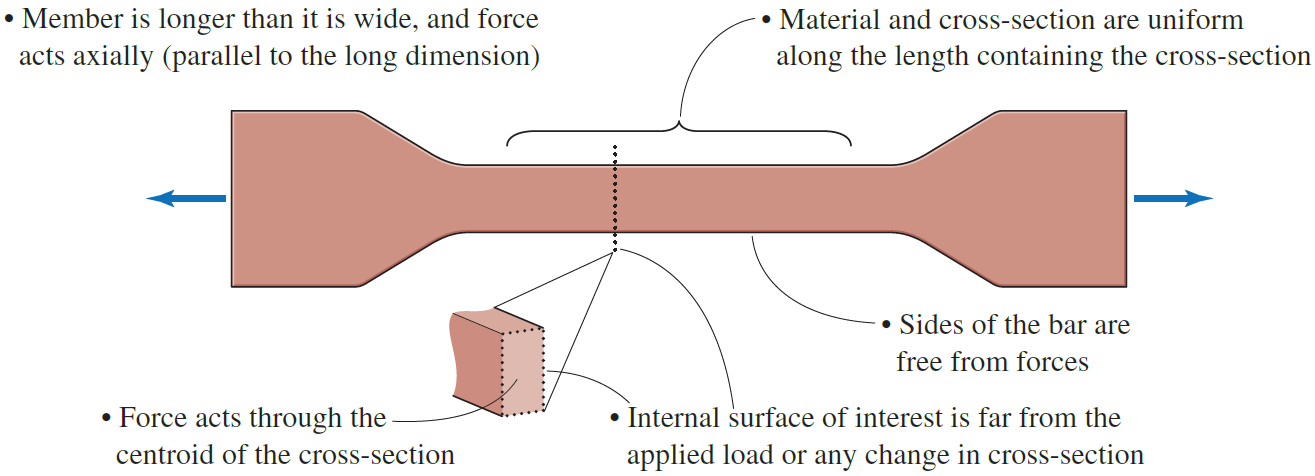St. Venant’s principle refers to the observation that the stress distribution becomes uniform as one moves away from the concentrated applied forces, subject to the above conditions.

7. Stresses that act to shorten an element are designated as compressive. Normal stresses shown so far, which elongate a body, are tensile. Normal stresses may act instead to shorten a body (compressive). Neighboring elements then press on each other, as in this structural post. Besides giving the magnitude of the normal stress, we either specify that the stress is tensile or compressive, or we use a positive sign to denote tension and negative to denote compression.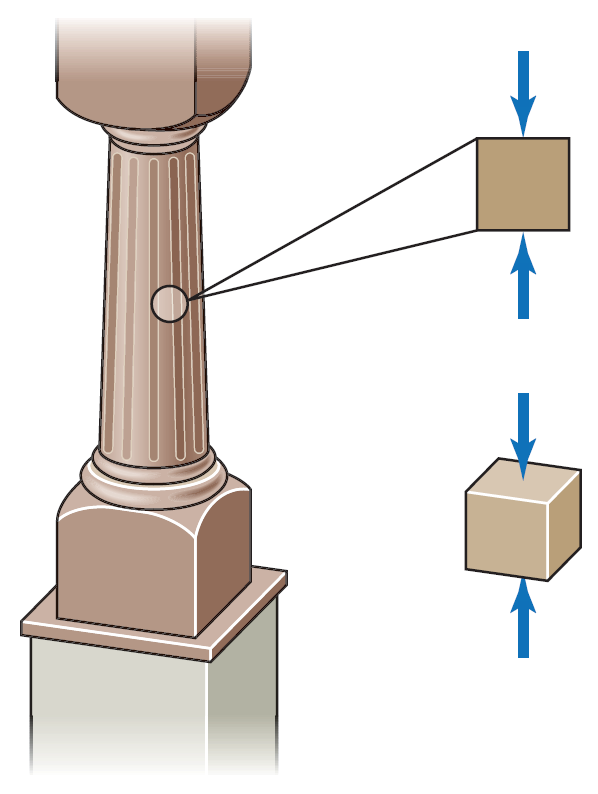Compressive stresses on a column
[blogger]

Engineeersdaily

Name

Email *

Message *